Open in App
Not now

# Applying a custom function on PySpark Columns with UDF

• Last Updated : 30 Jan, 2023

In this article, we are going to learn how to apply a custom function on Pyspark columns with UDF in Python.

The most useful feature of Spark SQL & DataFrame that is used to extend the PySpark build-in capabilities is known as UDF in Pyspark. There occurs various circumstances in which we need to apply a custom function on Pyspark columns. This can be achieved through various ways, but in this article, we will see how we can achieve applying a custom function on PySpark Columns with UDF.

Syntax: F.udf(function, T.column_type())

Parameters:

• function: It is the function that you want to apply on the Pyspark columns using UDF.
• column_type: It is the type of column which you want to assign after the function has been applied.

## Stepwise Implementation:

Step 1: First of all, import the required libraries, i.e., SparkSession, functions and types. The SparkSession library is used to create the session, while functions gives access to list of built-in functions available in Pyspark. The types give access to all the data types in Pyspark.

`from pyspark.sql import SparkSession`

Step 2: Now, create a spark session using the getOrCreate() function.

`spark_session = SparkSession.builder.getOrCreate()`

Step 3: Then, read the CSV file or create the data frame using the createDataFrame() function on which you want to apply a custom function on the columns using UDF.

```data_frame=csv_file = spark_session.read.csv('#Path of CSV file',
sep = ',', inferSchema = True, header = True)```

or

```data_frame=spark_session.createDataFrame([(column_1_data), (column_2_data), (column_3_data)],
['column_name_1', 'column_name_2', 'column_name_3'])```

Step 4: Later on, apply a custom function on PySpark Columns with UDF.

`custom_function = F.udf(function, T.column_type())`

Step 5: Further, create new columns by applying that function to the columns.

```updated_df = df.withColumn('column_1',
custom_function('column_1')).withColumn('column_2',
custom_function('column_2'))```

Step 6: Finally, display the updated data frame

`updated_df.show()`

### Example 1:

In this example, we have read the CSV file (link), i.e., basically a dataset of 5*5 as follows: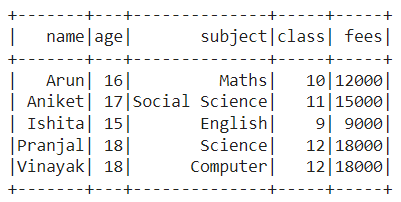Then, we calculated the current year and applied a custom function on Pyspark columns using UDF to calculate the birth year by subtracting age from the current year. Finally, we created a new column ‘Birth Year‘ by calling that function, i.e., birth_year, and displayed the data frame.

## Python3

 `# Python program to apply a custom  ` `# function on PySpark Columns with UDF ` ` `  `# Import the libraries SparkSession, functions, types and date ` `from` `pyspark.sql ``import` `SparkSession ` `import` `pyspark.sql.functions as F ` `import` `pyspark.sql.types as T ` `from` `datetime ``import` `date ` ` `  `# Create a spark session using getOrCreate() function ` `spark_session ``=` `SparkSession.builder.getOrCreate() ` ` `  `# Read the CSV file ` `df``=``csv_file ``=` `spark_session.read.csv(``'/content/student_data.csv'``, ` `              ``sep ``=` `','``, inferSchema ``=` `True``, ` `                         ``header ``=` `True``) ` ` `  `# Get current year ` `current_year``=``date.today().year ` ` `  `# Applying a custom function on PySpark Columns with UDF ` `birth_year ``=` `F.udf(``lambda` `age: current_year``-``age, ` `                   ``T.StringType()) ` ` `  `# Create new column for calculating the birth year ` `updated_df ``=` `df.withColumn(``'Birth Year'``, ` `                           ``birth_year(``'age'``)) ` ` `  `# Display the updated data frame ` `updated_df.show()`

Output: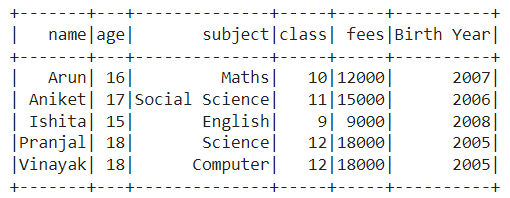### Example 2:

In this example, we have created the data frame with four columns, ‘name‘, ‘maths_marks‘, ‘science_marks‘, and ‘english_marks‘ as follows: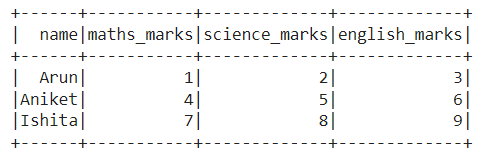Then, we applied a custom function to add all the marks on Pyspark columns using UDF and created a new column ‘Sum of Marks‘ by calling that function, i.e., sum_marks. Finally, displayed the data frame.

## Python3

 `# Python program to apply a custom  ` `# function on PySpark Columns with UDF ` ` `  `# Import the libraries SparkSession, functions, types ` `from` `pyspark.sql ``import` `SparkSession ` `import` `pyspark.sql.functions as F ` `import` `pyspark.sql.types as T ` ` `  `# Create a spark session using getOrCreate() function ` `spark_session ``=` `SparkSession.builder.getOrCreate() ` ` `  `# Create a data frame with duplicate column names ` `df ``=` `spark_session.createDataFrame( ` `  ``[(``'Arun'``,``1``,``2``,``3``),(``'Aniket'``,``4``,``5``,``6``), ` `                  ``(``'Ishita'``,``7``,``8``,``9``)], ` `  ``[``'name'``,``'maths_marks'``,``'science_marks'``, ` `                        ``'english_marks'``]) ` ` `  `# Applying a custom function on PySpark Columns with UDF ` `sum_marks``=``F.udf(``lambda` `a,b,c: a``+``b``+``c, ` `                         ``T.IntegerType()) ` ` `  `# Display the data frame with new column calling the  ` `# sum_marks function with marks as arguments ` `updated_df ``=` `df.withColumn(``'Sum of Marks'``, ` `             ``sum_marks(``'maths_marks'``,  ` `                       ``'science_marks'``, ` `                       ``'english_marks'``) ) ` ` `  `# Display the updated data frame ` `updated_df.show()`

Output: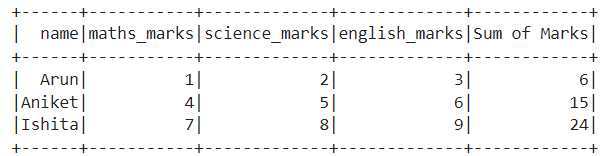### Example 3:

In this example, we have created the data frame with four columns, ‘name‘, ‘maths_marks‘, ‘science_marks‘, and ‘english_marks‘ as follows:Then, we applied a custom function to calculate the percentage of all the marks on Pyspark columns using UDF and created a new column ‘Percentage‘ by calling that function, i.e., percentage. Finally, displayed the data frame.

## Python3

 `# Python program to apply a custom  ` `# function on PySpark Columns with UDF ` ` `  `# Import the libraries SparkSession, functions, types ` `from` `pyspark.sql ``import` `SparkSession ` `import` `pyspark.sql.functions as F ` `import` `pyspark.sql.types as T ` ` `  `# Create a spark session using getOrCreate() function ` `spark_session ``=` `SparkSession.builder.getOrCreate() ` ` `  `# Create a data frame with duplicate column names ` `df ``=` `spark_session.createDataFrame( ` `  ``[(``'Arun'``,``1``,``2``,``3``),(``'Aniket'``,``4``,``5``,``6``), ` `                  ``(``'Ishita'``,``7``,``8``,``9``)], ` `  ``[``'name'``,``'maths_marks'``,``'science_marks'``, ` `                        ``'english_marks'``]) ` ` `  `# Applying a custom function on PySpark Columns with UDF ` `percentage``=``F.udf(``lambda` `a,b,c: ((a``+``b``+``c)``/``30``)``*``100``,  ` `                                   ``T.FloatType()) ` ` `  `# Display the data frame with new column calling the ` `# percentage function with marks as arguments ` `updated_df ``=` `df.withColumn(``'Percentage'``, ` `             ``percentage(``'maths_marks'``, ``'science_marks'``, ` `                                       ``'english_marks'``) ) ` ` `  `# Display the updated data frame ` `updated_df.show()`

Output: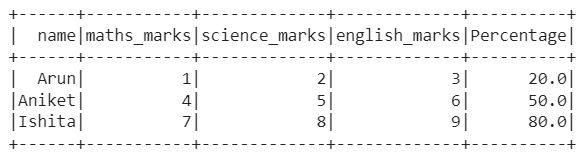My Personal Notes arrow_drop_up
Related Articles